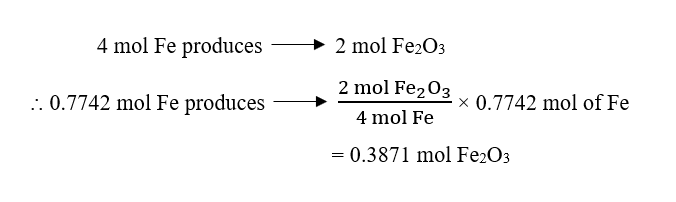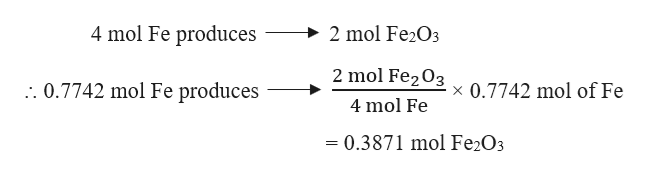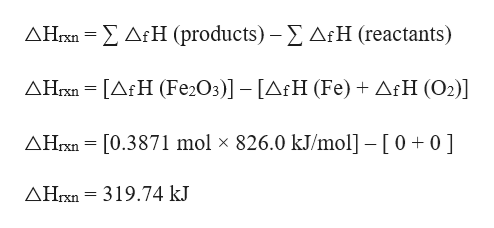# The heat of formation of Fe2O3(s) is 826.0 kJ/mol. Calculate the heat of the reaction when a 43.20 g sample of iron is reacted. a. 159.7 kJb. 1.784 × 104 kJc. 638.9 kJd. 1278 kJe. 319.5 kJ thanks for your help! :)

Question
11 views
The heat of formation of Fe2O3(s) is 826.0 kJ/mol. Calculate the heat of the reaction when a 43.20 g sample of iron is reacted.

a. 159.7 kJ
b. 1.784 × 104 kJ
c. 638.9 kJ
d. 1278 kJ
e. 319.5 kJ

check_circle

star
star
star
star
star
1 Rating
Step 1

Given,

Heat of formation of Fe2O3 = 826.0 kJ/mol

Mass of iron, Fe = 43.20 g

Moles of Fe can be calculated as:

Step 2

The balanced chemical reaction for the formation of Fe2O3 from iron, Fe can be written as :4 Fe (s) + 3 O2 (g) → 2 Fe2O3 (s)

From the given reaction, it is evident that :help_outlineImage Transcriptionclose4 mol Fe produces 2 mol Fe2O3 2 mol Fe203 .. 0.7742 mol Fe produces x 0.7742 mol of Fe 4 mol Fe = 0.3871 mol Fe2O3 fullscreen
Step 3

The standard enthalpies of formation are:

△f H (Fe) = 0 kJ/mol

△f H (O2) = 0 kJ/mol

△f H (Fe2O3) = 826.0 kJ/mol

The hea...help_outlineImage TranscriptioncloseΔΗΣ Δ:Η (products) -Σ ΔrΗ (reactants) ΔΗΚ- [ΔtΗ (Fe2O:)]-[AΗ (Fe) + ΔιΗ (Ο2)] ΔΗΚ[0.3871 mol x 826.0 kJmol] -[ 0+0 ] ΔΗΚ- 319.74 kJ fullscreen

### Want to see the full answer?

See Solution

#### Want to see this answer and more?

Solutions are written by subject experts who are available 24/7. Questions are typically answered within 1 hour.*

See Solution
*Response times may vary by subject and question.
Tagged in

### Chemical Thermodynamics